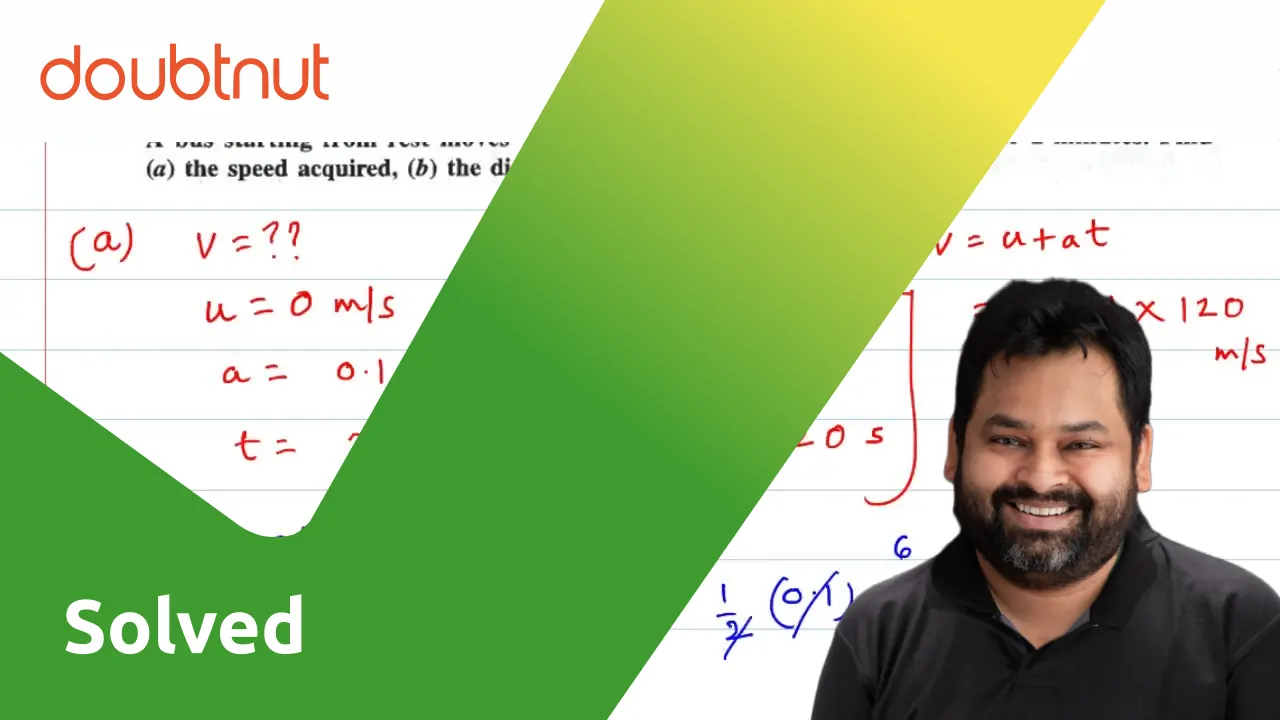Doubtnut App - Get 50 Lakh+ video solutions better than GoogleInstall Now

# A bus starting from rest moves with a uniform acceleration of 0.1m/s2 for 2 minutes. Find (a) the speed acquired, (b) th distance travelled.

Text Solution
Verified by Experts

## Here, u=0, a=0.1m/s2, t=2min=2×60s=120s speed acquired, v=? distance travelled, s=? (a) From v=u+at, v=0+0.1×120=12m/s (b) From s=ut+12at2, s=0×120+12×0.1(120)2=7.2×102m